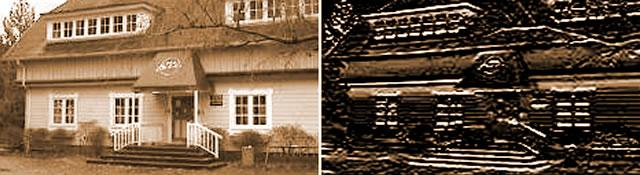## The Sobel and Laplacian Edge Detectors

Detecting edges is one of the fundamental operations you can do in image processing. It helps you reduce the amount of data (pixels) to process and maintains the "structural" aspect of the image. We'll look at two  commonly used edge detection schemes - the gradient based edge detector and the laplacian based edge detector. Both of them work with convolutions and achieve the same end goal - finding edges.

## Relating edges and derivatives

Just for this section, I'll limit the discussion to one dimensional images. It's difficult to "show" it if the things are in two dimensions. Have a look at this:

The lower part is the 1-D image. The upper part is the intensity of each pixel of the 1-D image plotted as a graph. Blacks have a low intensity, so the graph curve is low. It reaches full height at the white end of the image. Note that the center of the curve has a steep slope - meaning you've got an edge!

Looking for these peaks is exactly what gradient based edge detection methods do. You're not interested in what the actual colors are. If the change is steep enough, you mark it as an edge pixel. Though these methods work well, there's one drawback - how do you decide what is a peak and what isn't? There has to be a certain threshold above which an edge is classified as a peak else it must be considered part of noise. Here's the next key idea: On the left (where the curve is rising), the slope is positive. On the right, the slope is negative. So there must exist a point where there is a zero crossing. That point is the edge's location. Edge detectors that are based on this idea are called Laplacian edge detectors.

Now, all of this is for 1-D images. It turns out that all of this holds for 2-D images as well. So we can simply use these results and try them on actual images. Another thing is - these are based on continuous images. For us, that is never the case. So we'll have to approximate these derivatives based on the pixelated data that we do have. This is done with the help of convolutions.

## The Sobel Edge Detector

The Sobel edge detector is a gradient based method. It works with first order derivatives. It calculates the first derivatives of the image separately for the X and Y axes. The derivatives are only approximations (because the images are not continuous). To approximate them, the following kernels are used for convolution:

The kernel on the left approximates the derivative along the X axis. The one on the right is for the Y axis. Using this information, you can calculate the following:

• Magnitude or "strength" of the edge:• Approximate strength:• The orientation of the edge:Above, I've detected horizontal peaks. You can clearly see the horizontal edges highlighted. You can then threshold this result to get rid of the grey areas and get solid edges.

## The Laplacian Edge Detector

Unlike the Sobel edge detector, the Laplacian edge detector uses only one kernel. It calculates second order derivatives in a single pass. Here's the kernel used for it:

You can use either one of these. Or if you want a better approximation, you can create a 5x5 kernel (it has a 24 at the center and everything else is -1). Simple stuff. One serious drawback though - because we're working with second order derivatives, the laplacian edge detector is extremely sensitive to noise. Usually, you'll want to reduce noise - maybe using the Gaussian blur. Here's a result I got:

Laplacians are computationally faster to calculate (only one kernel vs two kernels) and sometimes produce exceptional results!

#### Related posts#### 7 unique neural network architectures